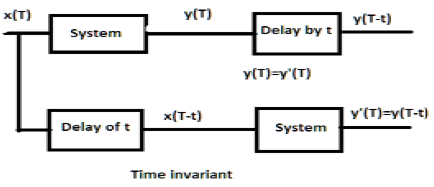# DSP - Time-Invariant Systems

#### Digital Marketing Online Training

23 Lectures 2 hours

#### How To Start Your Own Digital Marketing Agency

125 Lectures 9 hours

#### Digital Marketing Agency Elite Consultants Masterclass

140 Lectures 20 hours

For a time-invariant system, the output and input should be delayed by some time unit. Any delay provided in the input must be reflected in the output for a time invariant system.## Examples

a) $y(T) = x(2T)$

If the above expression, it is first passed through the system and then through the time delay (as shown in the upper part of the figure); then the output will become $x(2T-2t)$. Now, the same expression is passed through a time delay first and then through the system (as shown in the lower part of the figure). The output will become $x(2T-t)$.

Hence, the system is not a time-invariant system.

b) $y(T) = \sin [x(T)]$

If the signal is first passed through the system and then through the time delay process, the output be $\sin x(T-t)$. Similarly, if the system is passed through the time delay first then through the system then output will be $\sin x(T-t)$. We can see clearly that both the outputs are same. Hence, the system is time invariant.